### Preamble

Here, we present worked examples of Continuous Density Hidden Markov Models (CDHMM). The wording “continuous density” refers to the emission probabilities connected to the hidden states. In the first example, the emission probabilities are assumed to be normally (Gaussian) distributed with known means and standard deviations. This implies that we are not going to infer these parameters, but we want to know which emitter is active at which time point. Note that we presuppose that exactly one of the emitters is radiating at any point in time. In another paragraph, we will use the Baum-Welch algorithm to optimize the parameters of the model, including means, standard deviations, and transition probabilities. Finally, in the last example, we are choosing another (non-Gaussian) emission profile.
Much attention has been paid to explain in some detail how the log-likelihood was calculated. However, this paragraph can be skipped if not of interest, without loosing comprehensibility of other parts of this document.

Theory, diction and labeling refer to the documents:
- The EM algorithm for Hidden Markov Models
- The Hidden Markov Model: transition and emission probabilities

### A Hidden Markov Model (HMM)

In order to run this example, we are going to use the HiddenMarkov library by David Harte:

library(HiddenMarkov)

To start, let us define the hidden states, the transition probabilities between the states, and the parameters of the Gaussians.

We assume that we have three emitters (“EmitterA”, “EmitterB”, “EmitterC”) which successively come into action. However, we can’t see what emitter is radiating in what instant - our challenge is to find this out based on the available observations. The latter is the strength of the emitted signal at each point in time. As already pointed out, we know the means (stored in vector “means”) and standard deviations (stored in vector “sds”) of the Gaussian emission probabilities:

states = c("EmitterA", "EmitterB", "EmitterC")
means = c(0, 3, 8)
sds = c(0.02, 0.06, 0.1)
emitters = data.frame(State = states, Mean = means, StDev = sds)
emitters
##      State Mean StDev
## 1 EmitterA    0  0.02
## 2 EmitterB    3  0.06
## 3 EmitterC    8  0.10

We don’t know in which state the system starts. Consequently, we assign the same start probabilities to all emitters (by specifying the vector “startProbs”).

startProbs = c(1/3,1/3,1/3) 

Finally, we assign transition probabilities between the states (matrix $$\alpha$$):

alpha = matrix(c(0.6,0.2,0.2,0.8,0.1,0.1,0.4,0.3,0.3), nrow = 3, byrow = TRUE)
rownames(alpha) = states
colnames(alpha) = states
alpha
##          EmitterA EmitterB EmitterC
## EmitterA      0.6      0.2      0.2
## EmitterB      0.8      0.1      0.1
## EmitterC      0.4      0.3      0.3

Below a plot of the model including the parameter set: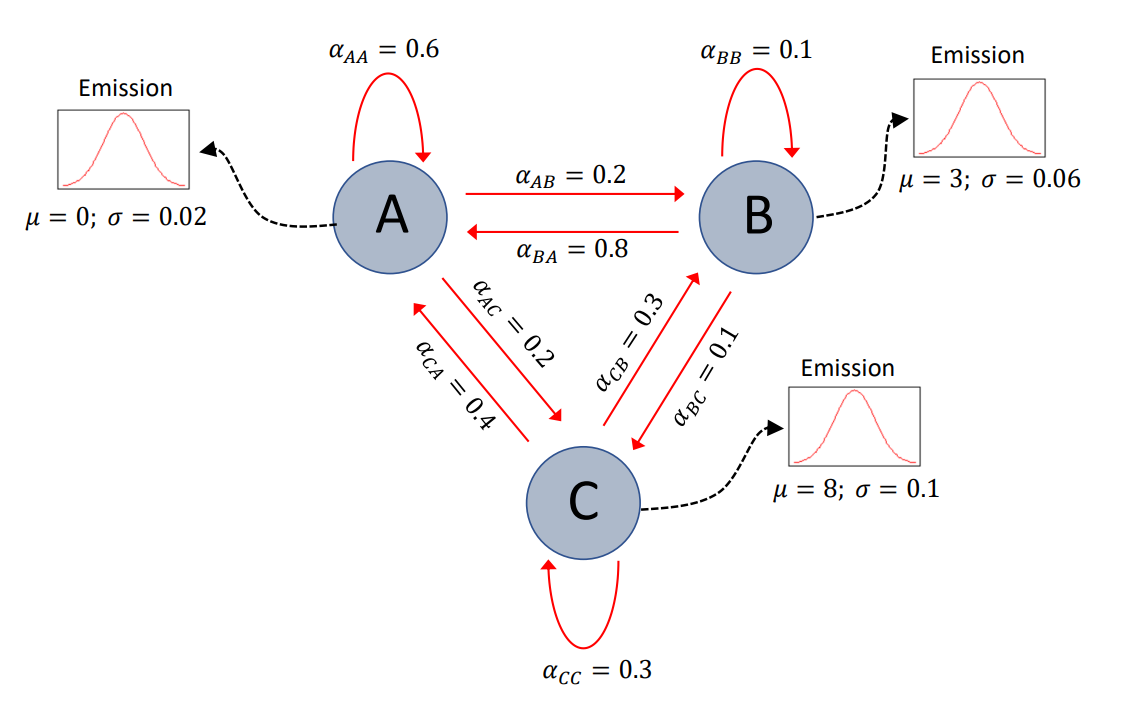We see the three emitters labelled “A”, “B”, and “C”, together with the transition- and emission probabilities. For example, we have the transition probability $$\alpha_{AA} = 0.6$$, indicating the probability that the system remains in state “A” in the subsequent time point when currently in “A”. On the other hand, $$\alpha_{AB} = 0.2$$ means that there is a 20% chance that the system jumps to state “B” at the next time point when currently in state “A”.
We have to admit that this should be an easy-to-solve problem, because the distances of the means of the Gaussian emitters are far apart compared to their standard deviations, so that the signal intensities should form very well separated blocks over time.

### Simulation of observations

Next, we simulate some observations. We let the emitters radiate normally distributed signals using the means and standard deviations defined above. We let the emitters come into play in the order “EmitterA”, “EmitterB”, “EmitterC”, “EmitterB”, “EmitterA”, that is 1-2-3-2-1, determined by the vector “order”. The span of the individual emissions is defined by the vector “duration”. As a matter of course, these parameters are not known to the algorithm that is supposed to uncover just these numbers. The numbers are only used to simulate the observations.

order = c(1,2,3,2,1)              # emitters are active in this order
duration = c(40, 50, 60, 50, 30)  # emitters are active for this period of time
signal = data.frame(State = emitters[order, "State"], Duration = duration)
signal
##      State Duration
## 1 EmitterA       40
## 2 EmitterB       50
## 3 EmitterC       60
## 4 EmitterB       50
## 5 EmitterA       30

Now, we generate the Gaussian signals using the above defined means, standard deviations, and durations, in order to get the signal strength over time:

set.seed(777)
obs = list()
for (i in 1:length(order)) obs[[i]] = rnorm(duration[i], mean = means[order[i]], sd = sds[order[i]])
observation = unlist(obs)
length(observation)  # 230 time points
##  230

Here comes a plot of the simulated signal:

colors = c("red", "blue", "darkgreen")
colvecpart = list()
for (i in 1:length(order)) colvecpart[[i]] = rep(colors[order[i]], duration[i])
colvec = unlist(colvecpart)

plot(1:length(observation), observation, col = colvec, type = "p", xlab = "time",
ylab = "signal", pch = 16, cex = 0.85)
title("Emitted signal over time", font.main = 1)
txt = c(emitters[1, "State"], emitters[2, "State"], emitters[3, "State"])
legend("topleft", txt, col = colors, lwd = 3, cex = 0.8)
mtext("Normally distributed signal", side = 3, col = "blue")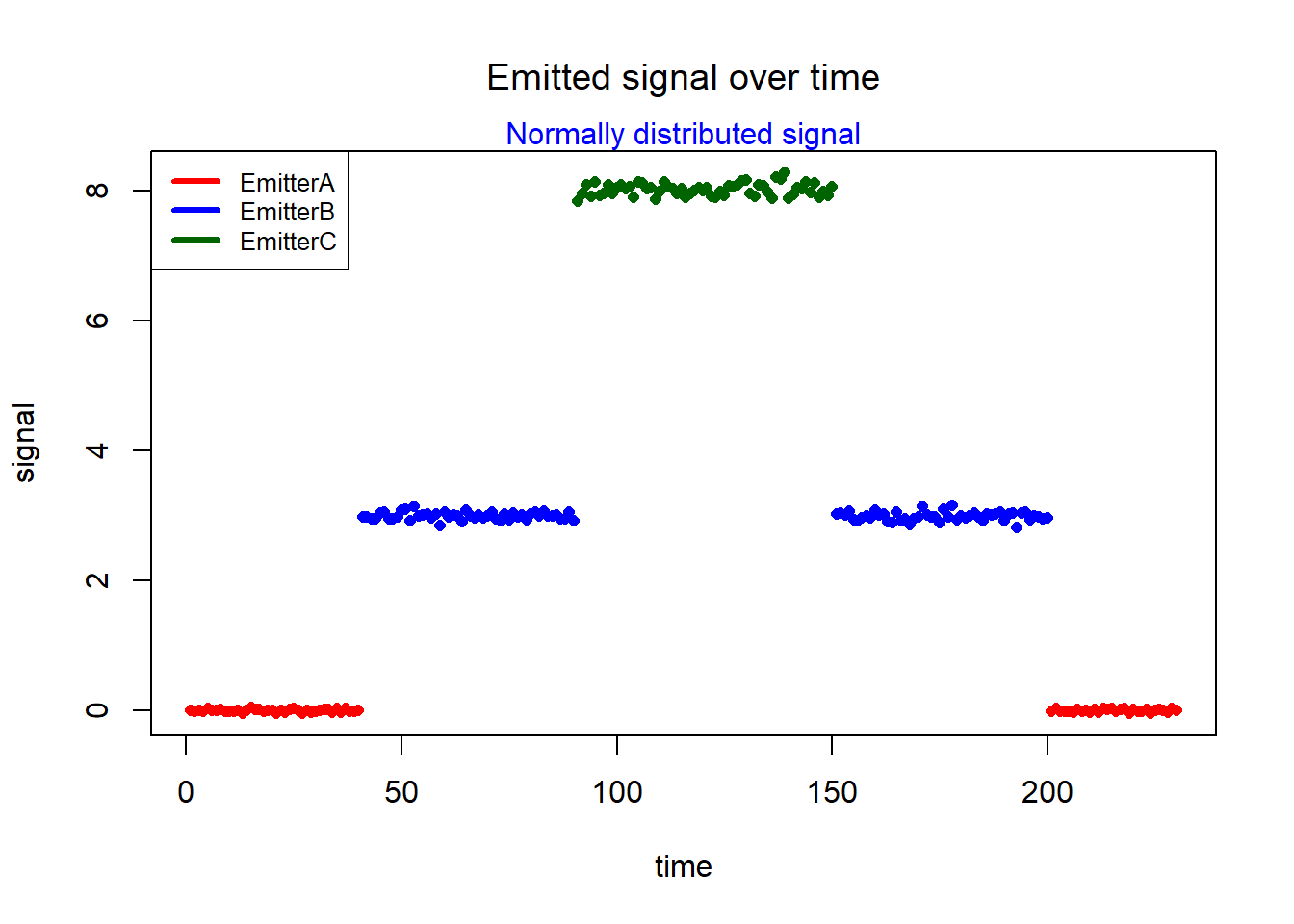We store the true state path, just to compare the calculated results with these true values later:

true_state_path = list()
for (i in 1:length(order)) true_state_path[[i]] = rep(order[i], duration[i])
true_state_path = unlist(true_state_path)
length(true_state_path)
##  230
true_state_path
##    1 1 1 1 1 1 1 1 1 1 1 1 1 1 1 1 1 1 1 1 1 1 1 1 1 1 1 1 1 1 1 1 1 1 1 1 1
##   1 1 1 2 2 2 2 2 2 2 2 2 2 2 2 2 2 2 2 2 2 2 2 2 2 2 2 2 2 2 2 2 2 2 2 2 2
##   2 2 2 2 2 2 2 2 2 2 2 2 2 2 2 2 3 3 3 3 3 3 3 3 3 3 3 3 3 3 3 3 3 3 3 3 3
##  3 3 3 3 3 3 3 3 3 3 3 3 3 3 3 3 3 3 3 3 3 3 3 3 3 3 3 3 3 3 3 3 3 3 3 3 3
##  3 3 2 2 2 2 2 2 2 2 2 2 2 2 2 2 2 2 2 2 2 2 2 2 2 2 2 2 2 2 2 2 2 2 2 2 2
##  2 2 2 2 2 2 2 2 2 2 2 2 2 2 2 1 1 1 1 1 1 1 1 1 1 1 1 1 1 1 1 1 1 1 1 1 1
##  1 1 1 1 1 1 1 1

In the vector “true_state_path”, “1” means “EmitterA”, “2” means “EmitterB”, and “3” means “EmitterC”.

### Finding the most likely state path - Viterbi algorithm

Some theory regarding the Viterbi algorithm was presented in Lecture 3 above.

In order to use the package HiddenMarkov, we have to define a list including the parameters of the emission function. Because we use a normal distribution, this list must coincide with those used by the functions dnorm or rnorm, which are mean and sd, see the HiddenMarkov manual.
Here, we store the parameters in the vector “eparams”:

eparams = list(mean = means, sd = sds)
eparams
## $mean ##  0 3 8 ## ##$sd
##  0.02 0.06 0.10

Moreover, we have to use the function dthmm in order to create a discrete time hidden Markov model object with class “dthmm”:

model = dthmm(x = observation, Pi = alpha, delta = startProbs, distn = "norm", pm = eparams)
class(model) 
##  "dthmm"

The object includes all necessary data. Have a look at the HiddenMarkov manual for details concerning the dthmm object, or run the command “str(model)” in R in order to see the components of the object.

Now, we are able to decode the observation by applying the Viterbi algorithm. This is done using the function “Viterbi” included in the package. The only argument needed by this function is the dthmm object created above:

states_predicted <- Viterbi(model)
states_predicted
##    1 1 1 1 1 1 1 1 1 1 1 1 1 1 1 1 1 1 1 1 1 1 1 1 1 1 1 1 1 1 1 1 1 1 1 1 1
##   1 1 1 2 2 2 2 2 2 2 2 2 2 2 2 2 2 2 2 2 2 2 2 2 2 2 2 2 2 2 2 2 2 2 2 2 2
##   2 2 2 2 2 2 2 2 2 2 2 2 2 2 2 2 3 3 3 3 3 3 3 3 3 3 3 3 3 3 3 3 3 3 3 3 3
##  3 3 3 3 3 3 3 3 3 3 3 3 3 3 3 3 3 3 3 3 3 3 3 3 3 3 3 3 3 3 3 3 3 3 3 3 3
##  3 3 2 2 2 2 2 2 2 2 2 2 2 2 2 2 2 2 2 2 2 2 2 2 2 2 2 2 2 2 2 2 2 2 2 2 2
##  2 2 2 2 2 2 2 2 2 2 2 2 2 2 2 1 1 1 1 1 1 1 1 1 1 1 1 1 1 1 1 1 1 1 1 1 1
##  1 1 1 1 1 1 1 1

The vector “states_predicted” contains the hidden states predicted by the Viterbi algorithm.

Let us compare the prediction with the true states stored above. In order to do so, we can plot the true states together with the prediction:

plot(1:length(true_state_path), true_state_path, xlab = "time", ylab = "states", col = "red", pch = 18)
title("True states and predicted states", font.main = 1)
mtext("Prediction by Viterby", col = "blue")
points(1:length(true_state_path), states_predicted + 0.03, col = "blue", pch = 16, cex = 0.8)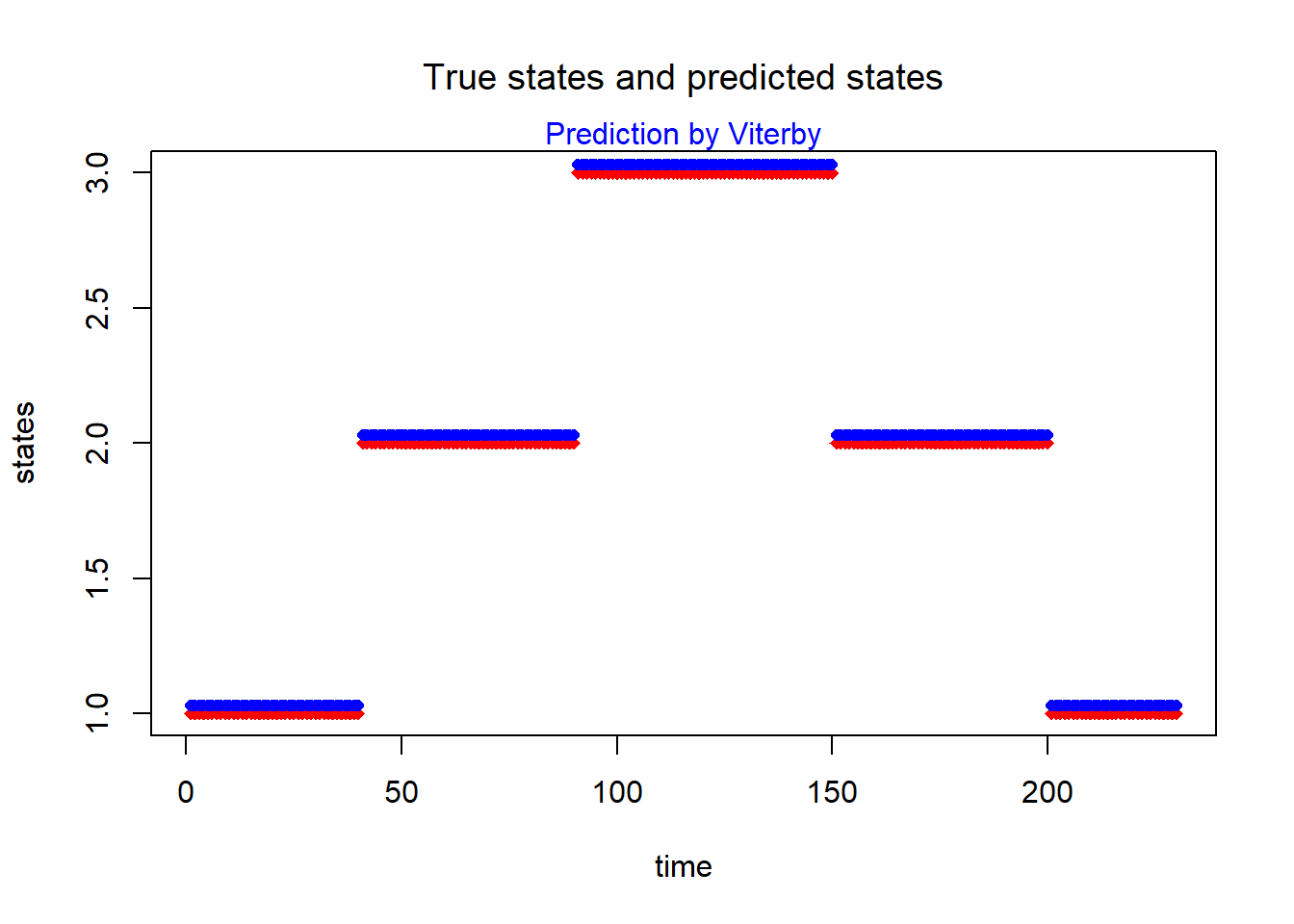Here, the predicted values have been artificially shifted 0.03 units upwards so that the true values are not completely covered. We see that there is perfect agreement between the true values and the prediction.

### A little harder problem

As already mentioned, this was an easy-to-solve problem, because the means of the emitters were distant compared to their standard deviations. Let’s make the challenge a little bit harder for the Viterbi by repeating the whole procedure with different data. You can also consider this as a summary of the essential commands:

states = c("EmitterA", "EmitterB", "EmitterC")
means = c(2, 3, 4)
sds = c(0.2, 0.3, 0.3)
emitters = data.frame(State = states, Mean = means, StDev = sds)
emitters
##      State Mean StDev
## 1 EmitterA    2   0.2
## 2 EmitterB    3   0.3
## 3 EmitterC    4   0.3
startProbs = c(1/3,1/3,1/3) 
alpha = matrix(c(0.6,0.2,0.2,0.8,0.1,0.1,0.4,0.3,0.3), nrow = 3, byrow = TRUE)
rownames(alpha) = states
colnames(alpha) = states
alpha
##          EmitterA EmitterB EmitterC
## EmitterA      0.6      0.2      0.2
## EmitterB      0.8      0.1      0.1
## EmitterC      0.4      0.3      0.3
order = c(1,2,3,2,1)              # emitters are active in this order
duration = c(40, 50, 60, 50, 30)  # emitters are active for this period of time
signal = data.frame(State = emitters[order, "State"], Duration = duration)
signal
##      State Duration
## 1 EmitterA       40
## 2 EmitterB       50
## 3 EmitterC       60
## 4 EmitterB       50
## 5 EmitterA       30
set.seed(777)
obs = list()
for (i in 1:length(order)) obs[[i]] = rnorm(duration[i], mean = means[order[i]], sd = sds[order[i]])
observation = unlist(obs)
length(observation)  # 230 time points
##  230

Keep in mind that you get different values in repeated runs of “rnorm” if you don’t set a seed.
Plot the simulated observation:

colors = c("red", "blue", "darkgreen")
colvecpart = list()
for (i in 1:length(order)) colvecpart[[i]] = rep(colors[order[i]], duration[i])
colvec = unlist(colvecpart)

plot(1:length(observation), observation, col = colvec, type = "p", xlab = "time", ylab = "signal",
pch = 16, cex = 0.85)
title("Emitted signal over time", font.main = 1)
txt = c(emitters[1, "State"], emitters[2, "State"], emitters[3, "State"])
legend("topleft", txt, col = colors, lwd = 3, cex = 0.8)
mtext("Normally distributed signal", side = 3, col = "blue")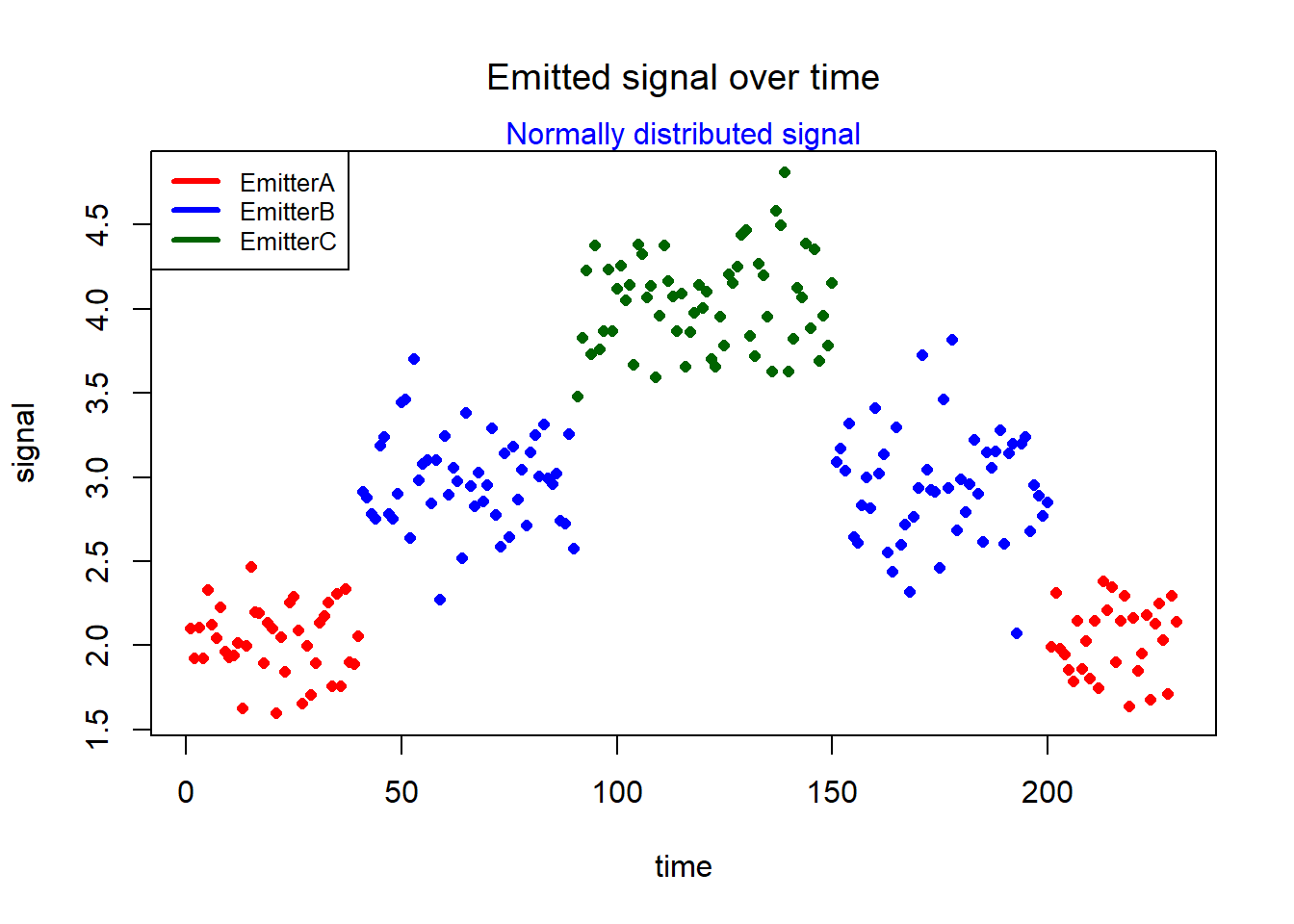The simulated signal shown in the above plot is definitely not that nice this time. Let’s see what the “Viterbi” can do:

eparams = list(mean = means, sd = sds)
eparams
## $mean ##  2 3 4 ## ##$sd
##  0.2 0.3 0.3
model = dthmm(x = observation, Pi = alpha, delta = startProbs, distn = "norm", pm = eparams)
class(model) 
##  "dthmm"
states_predicted <- Viterbi(model)
states_predicted
##    1 1 1 1 1 1 1 1 1 1 1 1 1 1 1 1 1 1 1 1 1 1 1 1 1 1 1 1 1 1 1 1 1 1 1 1 1
##   1 1 1 2 2 2 2 2 2 2 2 2 3 3 2 3 2 2 2 2 2 1 2 2 2 2 1 2 2 2 2 2 2 2 2 2 2
##   2 2 2 2 2 2 2 2 2 2 2 2 2 2 2 1 3 3 3 3 3 3 3 3 3 3 3 3 3 3 3 3 3 3 3 3 3
##  3 3 3 3 3 3 3 3 3 3 3 3 3 3 3 3 3 3 3 3 3 3 3 3 3 3 3 3 3 3 3 3 3 3 3 3 3
##  3 3 2 2 2 2 2 2 2 2 2 3 2 2 2 1 2 2 2 1 2 2 3 2 2 2 1 3 2 3 2 2 2 2 2 2 2
##  2 2 2 2 2 2 2 1 2 2 2 2 2 2 2 1 1 1 1 1 1 1 1 1 1 1 1 1 1 1 1 1 1 1 1 1 1
##  1 1 1 1 1 1 1 1
plot(1:length(true_state_path), true_state_path, xlab = "time", ylab = "states", col = "red", pch = 18)
title("True states and predicted states", font.main = 1)
mtext("Prediction by Viterby", col = "blue")
points(1:length(true_state_path), states_predicted + 0.03, col = "blue", pch = 16, cex = 0.8)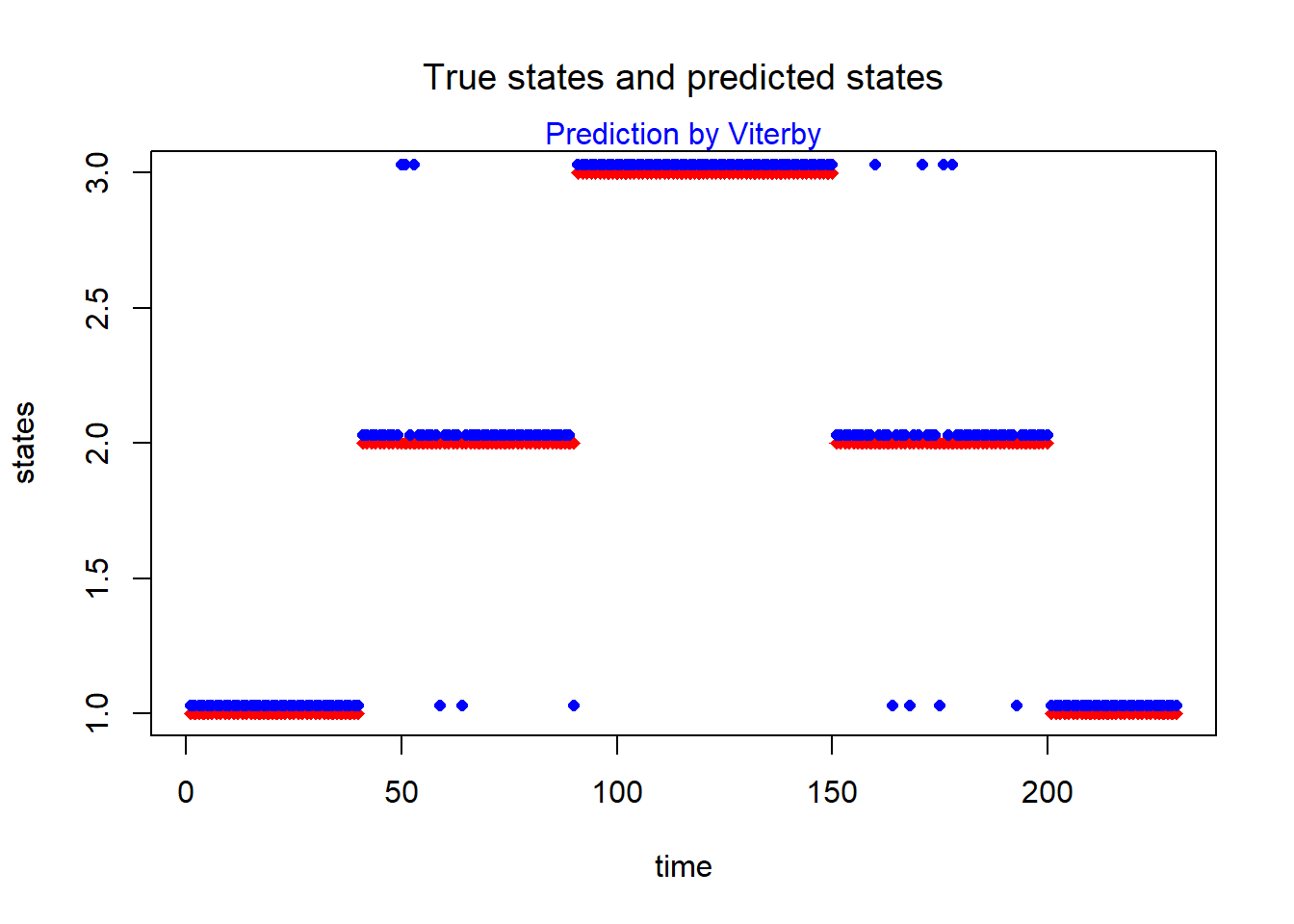Note that we have not repeated the definition of the vector containing the true state path. You can find the code further up. There a only about a dozen wrong predictions despite the rather irregular signal. Nice!

### Calculating the posterior probabilities

Another decoding approach is posterior decoding.
Here, we calculate the probability $$P \left( \pi_i = k \mid observation \right)$$, i.e. the probability that the $$i$$-th state in our state path is “EmitterA”, “EmitterB” or “EmitterC”, given the observed sequence. This can be calculated for all state path positions $$i$$. Note that this is different from the Viterbi approach. Posterior decoding was explained a little more in detail in Lecture 4 above. In the “HiddenMarkov” manual, posterior decoding is also called “local decoding”, in contrast to the expression “global decoding” which is used for the Viterbi algorithm. The Viterbi algorithm looks primarily at the sequence as a whole while posterior decoding focuses more on single positions in the state path.

The “HiddenMarkov” package provides the function “Estep” which can be employed to do this task. As a signal, we use the “irregular” observation lastly defined, stored in the vector “observation”. Here it is again:

colors = c("red", "blue", "darkgreen")
colvecpart = list()
for (i in 1:length(order)) colvecpart[[i]] = rep(colors[order[i]], duration[i])
colvec = unlist(colvecpart)

plot(1:length(observation), observation, col = colvec, type = "p", xlab = "time", ylab = "signal", pch = 18)
title("Emitted signal over time", font.main = 1)
txt = c(emitters[1, "State"], emitters[2, "State"], emitters[3, "State"])
legend("topleft", txt, col = colors, lwd = 3, cex = 0.8)
mtext("Normally distributed signal", side = 3, col = "blue")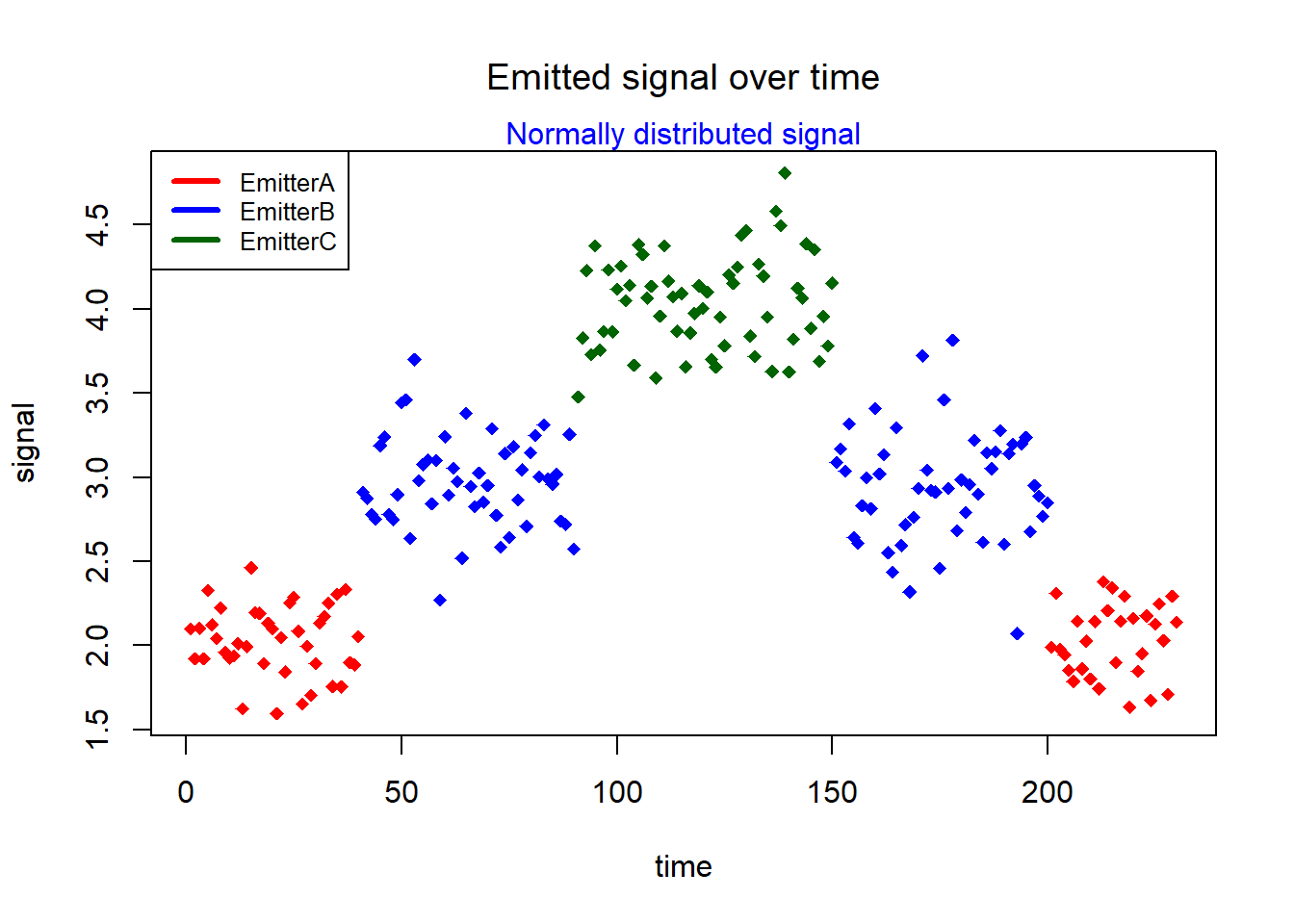The “Estep” function takes as arguments the observations, the transition probabilities, the start probabilities, a string indicating the distribution of the emission probabilities, and the parameters belonging to this distribution function, in this case means and standard deviations characterizing the normal distribution:

Estep_out = Estep(x = observation, Pi = alpha, delta = startProbs, distn = "norm", pm = eparams)
str(Estep_out)
## List of 3
##  $u : num [1:230, 1:3] 0.989 1 0.996 1 0.916 ... ##$ v : num [1:229, 1:3, 1:3] 0.989 0.996 0.996 0.915 0.911 ...
##  $LL: num -310 The posterior probabilities for each state and for each time point are included in the u-component of the Estep-output: postprob = Estep_out$u    # posterior probabilities
colnames(postprob) = states 

Let’s have a look a the first six time points:

head(postprob)   
##       EmitterA     EmitterB     EmitterC
## [1,] 0.9892133 0.0107866981 9.262114e-10
## [2,] 0.9995110 0.0004890130 5.868423e-12
## [3,] 0.9961845 0.0038154633 3.432922e-10
## [4,] 0.9995338 0.0004662075 6.475745e-12
## [5,] 0.9159056 0.0840942957 9.355932e-08
## [6,] 0.9952242 0.0047758426 5.505910e-10

We see that the probability is much bigger for “EmitterA” for these time points, so that “EmitterA” can be regarded as the posterior prediction for these time points. In order to get posterior predictions for the whole sequence, we have to identify the column with the biggest probability in each row. Here we go:

maxrow = apply(Estep_out$u, 1, which.max) maxrow ##  1 1 1 1 1 1 1 1 1 1 1 1 1 1 1 1 1 1 1 1 1 1 1 1 1 1 1 1 1 1 1 1 1 1 1 1 1 ##  1 1 1 2 2 2 2 2 2 2 2 2 3 3 2 3 2 2 2 2 2 1 2 2 2 2 1 2 2 2 2 2 2 2 2 2 2 ##  2 2 2 2 2 2 2 2 2 2 2 2 2 2 2 2 3 3 3 3 3 3 3 3 3 3 3 3 3 3 3 3 3 3 3 3 3 ##  3 3 3 3 3 3 3 3 3 3 3 3 3 3 3 3 3 3 3 3 3 3 3 3 3 3 3 3 3 3 3 3 3 3 3 3 3 ##  3 3 2 2 2 2 2 2 2 2 2 3 2 2 2 1 2 2 2 1 2 2 3 2 2 2 1 3 2 3 2 2 2 2 2 2 2 ##  2 2 2 2 2 2 2 1 2 2 2 2 2 2 2 1 1 1 1 1 1 1 1 1 1 1 1 1 1 1 1 1 1 1 1 1 1 ##  1 1 1 1 1 1 1 1 The associated posterior probabilities can simply be fetched with the “max” command: maxprob = apply(Estep_out$u, 1, max)
head(maxprob)
##  0.9892133 0.9995110 0.9961845 0.9995338 0.9159056 0.9952242

We have shown the first six probabilities only (compare with the vector “postprob” above!).

We can combine these findings in a data frame:

posterior = data.frame(State = maxrow, Prob = maxprob)
head(posterior)
##   State      Prob
## 1     1 0.9892133
## 2     1 0.9995110
## 3     1 0.9961845
## 4     1 0.9995338
## 5     1 0.9159056
## 6     1 0.9952242

Here comes a comparison of the predictions based on posterior probabilities with the true path:

plot(1:length(true_state_path), true_state_path, xlab = "time", ylab = "states", col = "red", pch = 18)
title("True states and predicted states", font.main = 1)
mtext("Posterior probabilities", col = "blue")
points(1:length(true_state_path), posterior$State + 0.03, col = "blue", pch = 16, cex = 0.8)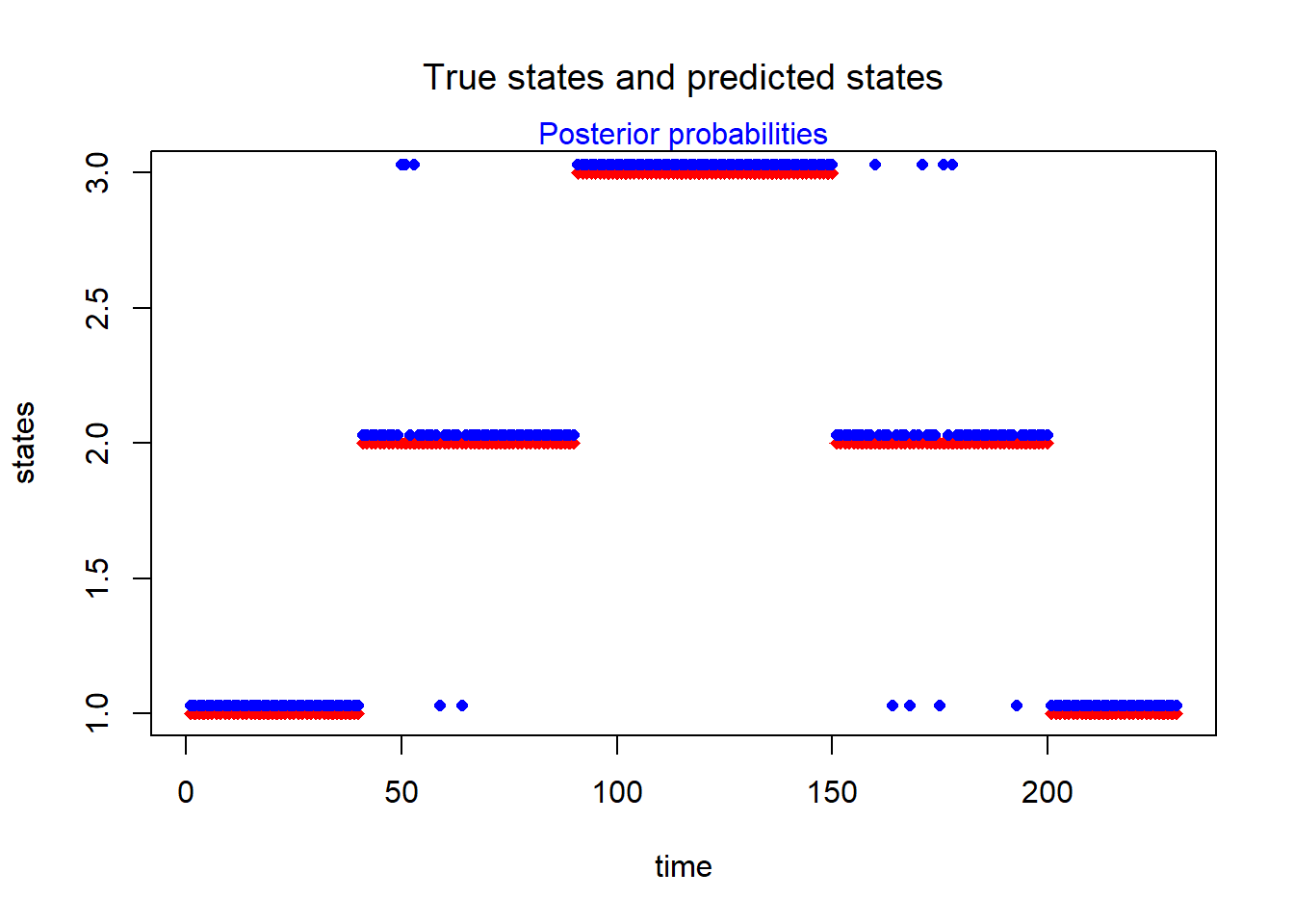Again, we have a few discrepancies only. ### Calculation of the Log-likelihood (This section can be skipped when not of interest, it contains quite a lot of statistical details …) #### Log-likelihood for a mixture of distribution functions Another component of the “Estep” output is the log-likelihood: Estep_out$LL
##  -309.6714

Here is what Wikipedia says about the likelihood when continuous probability distributions are involved:
“Let $$X$$ be a random variable … with density function $$f(x)$$ which depends on a parameter $$\theta$$. Then the function $\mathcal{L}\left( \theta \mid x \right) =f(x \mid \theta)$ considered as a function of $$\theta$$ is the likelihood function …”.

On the other hand, if we have a mixture of continuous distributions, the likelihood becomes a weighted sum of the density functions. If we have a mixture of three distributions, this reads:

$\mathcal{L}\left( \theta \mid x \right) = \lambda_1 \cdot f(x \mid \theta_1) + \lambda_2 \cdot f(x \mid \theta_2) + \lambda_3 \cdot f(x \mid \theta_3)$ where the weights add to one: $$\lambda_1 + \lambda_2 +\lambda_3 = 1$$. If we consider 3 normal distributions, we get:

$\mathcal{L}\left( \theta \mid x \right) = \lambda_1 \cdot \mathcal{N}(x \mid \mu_1 , \sigma_1) + \lambda_2 \cdot \mathcal{N}(x \mid \mu_2 , \sigma_2) + \lambda_3 \cdot \mathcal{N}(x \mid \mu_3 , \sigma_3)$ This is the formula we’ll need very soon (because we have 3 states with Gaussian emission profiles).

Note that likelihood is not a probability (it is a function of a parameter $$\theta$$, and the integral over $$\theta$$ is not one!) but it can be used to compare the plausibility of different parameter settings in statistical models. The bigger the likelihood, the more likely it is that the parameters underlying the model are those parameters that really generated the observed data. Read more about likelihood in this interesting article by Ajay Halthor.
Let us have a closer look at the log-likelihood of the above defined Hidden Markov Model by trying to recap how it was calculated. In order to do so, we have to calculate the likelihood of all observations throughout the whole timeline. While the likelihood value for the whole chain is obtained by multiplying the likelihood values referring to the individual time points, the log-likelihood values have to be added.

It is convenient to have the emission density values of all emitters at all time points at hand. These follow from the observations, as well as from the means and standard deviations of emitters A, B, and C:

epsilon = matrix(as.double(0), nrow = length(observation), ncol = ncol(alpha))
for (k in 1:length(means)) epsilon[, k] = dnorm(observation, mean = means[k], sd = sds[k])
colnames(epsilon) = states
rownames(epsilon) = paste0("x", 1:length(observation))
head(epsilon)  # emission densities
##     EmitterA    EmitterB     EmitterC
## x1 1.7692471 0.014473781 2.483320e-09
## x2 1.8424234 0.002046825 4.877615e-11
## x3 1.7507119 0.015096095 2.714131e-09
## x4 1.8422246 0.002045496 4.871517e-11
## x5 0.5209263 0.107988746 2.380297e-07
## x6 1.6446189 0.018766422 4.312518e-09

#### Log-likelihood of the first observation

Now, it is quite easy to calculate the likelihood of the observation at time point one. According to the definition of the likelihood given above, it is the weighted sum of the density distribution functions of the emitters A, B, and C at this time point, where the weights are the probabilities referring to these states. The latter are the initial probabilities stored in “startProbs”, i.e. the vector $$\left[1/3 , 1/3, 1/3\right]$$.

Lhood = sum(startProbs * epsilon[1, ])
Lhood
##  0.5945736

This is the likelihood at time point 1. The log-likelihood is therefore

logL = log(Lhood)
logL
##  -0.5199107

As we will see very soon, we need also to calculate the posterior probabilities that the system was in state A, B, or C at time point 1, given the observation $$x_1$$, i.e. we need the conditional probabilities $$P\left(\pi_1 = A \mid x_1\right)$$, $$P\left(\pi_1 = B \mid x_1\right)$$, and $$P\left(\pi_1 = C \mid x_1\right)$$. They are different from “startProbs”, because “startProbs” is a pure guess, while the conditional probabilities take the observed data $$x_1$$ into account.
The posterior probability that the first emission originated from state A, $$P\left(\pi_1 = A \mid x_1\right)$$, can be estimated using the expression

\begin{align*} P\left( \pi_1 =A \mid x_1 \right) &= \frac{P\left( x_1 = A \right) \cdot \mathcal{N}\left( x_1, \mu_A, \sigma_A \right) }{P\left( x_1 = A \right) \cdot \mathcal{N}\left( x_1, \mu_A, \sigma_A \right) + P\left( x_1 = B \right) \cdot \mathcal{N}\left( x_1, \mu_B, \sigma_B \right) +P\left( x_1 = C \right) \cdot \mathcal{N}\left( x_1, \mu_C, \sigma_C \right) } \\\\ &= \frac{1/3 \cdot \mathcal{N}\left( x_1, \mu_A, \sigma_A \right) }{1/3 \cdot \mathcal{N}\left( x_1, \mu_A, \sigma_A \right) + 1/3 \cdot \mathcal{N}\left( x_1, \mu_B, \sigma_B \right) + 1/3 \cdot \mathcal{N}\left( x_1, \mu_C, \sigma_C \right) } \end{align*} Calculation of $$P\left(\pi_1 = B \mid x_1\right)$$ and $$P\left(\pi_1 = C \mid x_1\right)$$ is done in a similar way. The rationale behind the above formula might become understandable with the help of this plot (showing a mixture of only two Gaussians):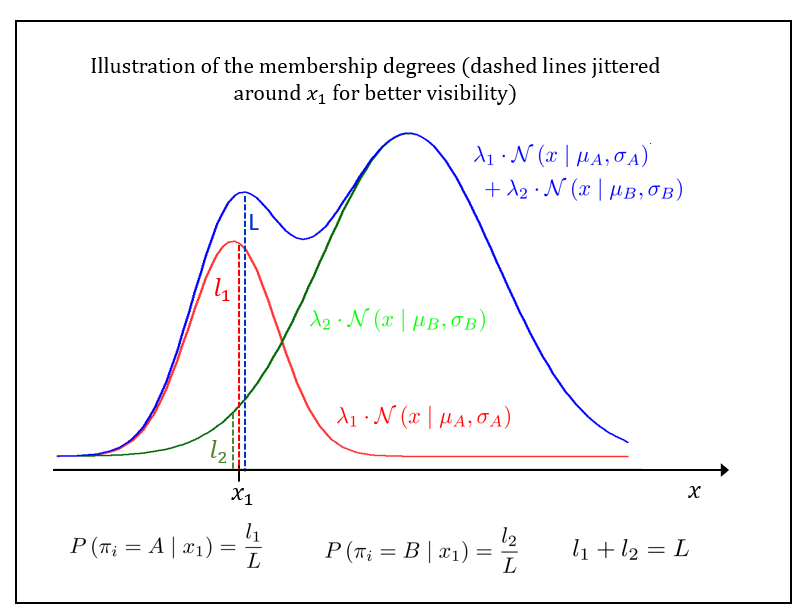We see that the posterior probability that an individual Gaussian generated the observation $$x_1$$ is estimated as the proportion with which this Gaussian contributes to the total signal intensity of $$x_1$$. A more detailed explanation of these so called “membership probabilities” can be found in this document or in the E-Step paragraph of this document.

In R, we calculate the posterior probabilities for state A, B, and C in the following way:

pi = startProbs * epsilon[1, ]/sum(startProbs * epsilon[1, ])
print(format(pi, digits = 3), quote = FALSE)
## EmitterA EmitterB EmitterC
## 9.92e-01 8.11e-03 1.39e-09

We see that it is most likely that the first emission came from emitter A. The vector $$\pi$$ contains probabilities and must therefore add up to 1:

sum(pi)
##  1

#### Log-likelihood of the second and all subsequent observations

In order to explain the observation $$x_2$$ at time point 2, we have to consider two events: a transition to the next state variable A, B, or C, and an emission from this state leading to the observation $$x_2$$.

The change of the state probabilities $$\pi$$ caused by the transition to the next time point can be calculated by multiplying the current probability vector $$\pi = \left[ P\left( \pi_1 = A \right) , P\left(\pi_1 = B \right) , P\left(\pi_1 = C \right) \right]$$ (conditioning on $$x_1$$ suppressed) with the transition matrix:

pi = pi %*% alpha
print(format(pi, digits = 3), quote = FALSE)
##      EmitterA EmitterB EmitterC
## [1,] 0.602    0.199    0.199

How can we motivate this?
Let’s have a look at the transition matrix, and what its elements mean in statistical terms:

$\alpha = \begin{bmatrix} P \left( \pi_2 = A \mid \pi_1 = A \right) & P\left( \pi_2 = B \mid \pi_1 = A \right) & P\left( \pi_2 = C \mid \pi_1 = A\right) \\ P \left( \pi_2 = A \mid \pi_1 = B \right) & P\left( \pi_2 = B \mid \pi_1 = B \right) & P\left( \pi_2 = C \mid \pi_1 = B \right) \\ P \left( \pi_2 = A \mid \pi_1 = C \right) & P\left( \pi_2 = B \mid \pi_1 = C \right) & P\left( \pi_2 = C \mid \pi_1 = C \right) \end{bmatrix}$

Therefore, the above R code multiplies

$\left[ P\left( \pi_1 = A \right) \;\;\; P\left(\pi_1 = B \right) \;\;\; P\left(\pi_1 = C \right) \right] \cdot \begin{bmatrix} P \left( \pi_2 = A \mid \pi_1 = A \right) & P\left( \pi_2 = B \mid \pi_1 = A \right) & P\left( \pi_2 = C \mid \pi_1 = A\right) \\ P \left( \pi_2 = A \mid \pi_1 = B \right) & P\left( \pi_2 = B \mid \pi_1 = B \right) & P\left( \pi_2 = C \mid \pi_1 = B \right) \\ P \left( \pi_2 = A \mid \pi_1 = C \right) & P\left( \pi_2 = B \mid \pi_1 = C \right) & P\left( \pi_2 = C \mid \pi_1 = C \right) \end{bmatrix}$

Let us only develop the first component $$p_1$$ of the result:

\begin{align*} p_1 &= P\left( \pi_1 = A \right) \cdot P \left( \pi_2 = A \mid \pi_1 = A \right) + P\left(\pi_1 = B \right) \cdot P \left( \pi_2 = A \mid \pi_1 = B \right) + P\left(\pi_1 = C \right) \cdot P \left( \pi_2 = A \mid \pi_1 = C \right) \\ &= P \left( \pi_1 = A , \pi_2 = A \right) + P \left( \pi_1 = B , \pi_2 = A \right) + P \left( \pi_1 = C , \pi_2 = A \right) \\ &= P \left(\pi_2 = A \right) \end{align*}

The last line follows from the marginal rule. Likewise, we can show that the $$2$$-nd and $$3$$-rd components are $$P\left(\pi_2 = B \right)$$ and $$P\left(\pi_2 = C \right)$$, respectively. To summarize, the above shown R code calculates the vector $$\left[ P\left(\pi_2 = A\right) , P\left(\pi_2 = B \right) , P \left(\pi_2 = C \right)\right]$$ , i.e. the state probabilities at time point 2.

We can check again if the state probabilities sum up to one:

sum(pi)
##  1

Now, as for observation $$x_1$$, we calculate the likelihood of the observation $$x_2$$ as a weighted sum of the density distribution functions of the emitters A, B, and C at this time point. Again, the weights are the actual state probabilities, stored in the just calculated vector $$\left[ P\left(\pi_2 = A\right) , P\left(\pi_2 = B \right) , P \left(\pi_2 = C \right)\right]$$.

In R code, this is

Lhood = sum(pi * epsilon[2, ])

The joint log-likelihood of observations one and two is therefore

logL = logL + log(Lhood)
logL
##  -0.4165857

(In order to get the joint likelihood of the chain, the likelihoods of all observations have to be multiplied, but the log-likelihoods must be added.)

Next, as for time point 1, we estimate the posterior state probabilities by calculating the membership degree of every emitter for observation $$x_2$$:

pi = pi * epsilon[2, ]/sum(pi * epsilon[2, ])
print(format(pi, digits = 3), quote = FALSE)
##      EmitterA EmitterB EmitterC
## [1,] 1.00e+00 3.68e-04 8.76e-12

Again, it might improve the trust in our algorithm if we can confirm that the calculated probabilities sum up to 1:

sum(pi)
##  1

Now, we repeat the steps carried out for time point 2 for all subsequent time points, until the end of the sequence is reached.

#### Summary of the algorithm to calculate the log-likelihood

To summarize, what we have done to get the log-likelihood is:

1. Calculate the emission density values of all emitters at all time points.
2. Get the likelihood of the $$1$$st observation by calculating a weighted sum of the emission densities of emitters A, B, and C.
3. Calculate the posterior state probabilities (given observation $$x_1$$).
4. Consider the transition to the next time point by multiplying the current state probability vector with the transition matrix. This yields new state probabilities.
5. Get the likelihood of the $$2$$nd observation by calculating a weighted sum of the emission densities referring to observation $$x_2$$.
6. Increment the log-likelihood value.
7. Calculate the posterior state probabilities (given the current observation).

Steps 4 to 7 are repeated until the end of the string of observations.

#### A function that calculates the log-likelihood

Based on the algorithm introduced above, the log-likelihood can be calculated using the following function:

logLikelihood <- function(observation, alpha, means, sds, startProbs) {
epsilon = matrix(as.double(0), nrow = length(observation), ncol = ncol(alpha))
for (k in 1:length(means)) epsilon[, k] = dnorm(observation, mean = means[k], sd = sds[k])

Lhood = sum(startProbs * epsilon[1, ])           # x_1
logL = log(Lhood)
pi = startProbs * epsilon[1, ]/sum(startProbs * epsilon[1, ])

for (i in 2:length(observation)) {               # x_2 until x_end
pi = pi %*% alpha                              # new state probabilities resulting from transition
Lhood = sum(pi * epsilon[i, ])                 # likelihood for next observation
logL = logL + log(Lhood)                       # increment log-likelihood
pi = pi * epsilon[i, ]/sum(pi * epsilon[i, ])  # new posterior state probabilities
}
out = list()
out[["epsilon"]] = epsilon
out[["logL"]] = logL
invisible(out)
}

The code presented is written for comprehensibility, not for efficiency. For instance, it is not efficient to calculate the expression $$sum(pi * epsilon[i, ])$$ in the loop twice. A faster approach would be to buffer the sum in a temporary variable. Moreover, it should be considered to assign all variables with double precision, because numerical errors may accumulate if the chain is long.

Finally, let us see if the function yields the same result as the output of the “Estep” function obtained above:

result = logLikelihood(observation, alpha, means, sds, startProbs)
result$logL  ##  -309.6714 The “Estep” result was: Estep_out$LL       
##  -309.6714

The log-likelihood can also be calculated using a function coming with the “HiddenMarkov” package. The only argument for this function is the “dthmm” object declared above:

logLik(model)   
##  -309.6714

### Finding the best model parameters - Baum-Welch algorithm

Above, the parameters of the model, e.g. the means and the standard deviations of the Gaussian emission distribution, as well as the transition probabilities, were given. However, this might not always be the case. Rather, it might rarely be the case. How can we now the model parameters underlying some observation? In order to unravel unknown parameters of an HMM, a variant of the Expectation-Maximization (EM) algorithm, the Baum-Welch algorithm, can be used.

Some details regarding EM and Baum-Welch were already provided in the article EM algorithm for Hidden Markov Models. An example for a mixture of three (2D-) Gaussians is presented here. You can also have a look at the “dishonest-casino” example.

The Baum-Welch algorithm finds maximum likelihood (ML) estimates of the HMM model parameters, given the observed emissions. It should be repeated here that the method finds local maximum values of the likelihood which might not be the global optimum (initial values of the parameters are critical).

Applying the Baum-Welch algorithm with the help of the HiddenMarkov package is rather simple. All we need is the model object defined above, which must be of class dthmm:

class(model)
##  "dthmm"

The behavior of the function can be tweaked using the bwcontrol variable. For details, see the HiddenMarkov manual. Having the model object at hand, we are ready to run the Baum-Welch:

bwout = BaumWelch(model, control = bwcontrol(maxiter = 500, tol = 1e-05, prt = FALSE))
str(bwout)
## List of 13
##  $x : num [1:230] 2.1 1.92 2.1 1.92 2.33 ... ##$ Pi      : num [1:3, 1:3] 9.85e-01 1.01e-02 1.81e-24 1.48e-02 9.80e-01 ...
##  $delta : num [1:3] 1.00 6.26e-22 2.51e-86 ##$ distn   : chr "norm"
##  $pm :List of 2 ## ..$ mean: num [1:3] 2.03 2.96 4.04
##   ..$sd : num [1:3] 0.207 0.303 0.275 ##$ pn      : NULL
##  $discrete: logi FALSE ##$ nonstat : logi TRUE
##  $u : num [1:230, 1:3] 1 1 1 1 1 ... ##$ v       : num [1:229, 1:3, 1:3] 1 1 1 1 1 ...
##  $LL : num -40.4 ##$ iter    : int 7
##  $diff : num 5.5e-06 ## - attr(*, "class")= chr "dthmm" The output of the BaumWelch function contains updated values of the HMM parameters. Let us compare the new parameters with the ones we had initially chosen: The new transition probabilities are stored in the Pi list element: print(format(bwout$Pi, digits = 3), quote = FALSE)
##      [,1]     [,2]     [,3]
## [1,] 9.85e-01 1.48e-02 1.27e-16
## [2,] 1.01e-02 9.80e-01 1.01e-02
## [3,] 1.81e-24 1.70e-02 9.83e-01

We had stored the original transition probabilities in the $$\alpha$$ matrix:

alpha
##          EmitterA EmitterB EmitterC
## EmitterA      0.6      0.2      0.2
## EmitterB      0.8      0.1      0.1
## EmitterC      0.4      0.3      0.3

We see that the numerical values of the parameters changed considerably.

Here are the new means and standard deviations of the Gaussian emission distribution, compared to the values we had choosen before:

bwout$pm$mean
##  2.027118 2.962835 4.044831
means
##  2 3 4
bwout$pm$sd 
##  0.2075000 0.3032232 0.2754277
sds  
##  0.2 0.3 0.3

Here, the differences are not too big.

Let us also have a look at the posterior probabilities (before and after Baum-Welch):

head(postprob)
##       EmitterA     EmitterB     EmitterC
## [1,] 0.9892133 0.0107866981 9.262114e-10
## [2,] 0.9995110 0.0004890130 5.868423e-12
## [3,] 0.9961845 0.0038154633 3.432922e-10
## [4,] 0.9995338 0.0004662075 6.475745e-12
## [5,] 0.9159056 0.0840942957 9.355932e-08
## [6,] 0.9952242 0.0047758426 5.505910e-10
head(bwout$u) ## [,1] [,2] [,3] ## [1,] 1.0000000 8.015430e-26 1.082473e-103 ## [2,] 0.9999997 3.311328e-07 3.104907e-35 ## [3,] 0.9999980 2.017395e-06 1.889249e-24 ## [4,] 0.9999996 4.039083e-07 8.131014e-24 ## [5,] 0.9999657 3.428319e-05 7.673936e-21 ## [6,] 0.9999968 3.154977e-06 9.792904e-22 As above, we can find the new posterior predictions for the whole sequence by identifying the column with the biggest probability in each row: maxrow = apply(bwout$u, 1, which.max)
maxprob = apply(bwout$u, 1, max) new_posterior = data.frame(State = maxrow, Prob = maxprob) head(new_posterior) ## State Prob ## 1 1 1.0000000 ## 2 1 0.9999997 ## 3 1 0.9999980 ## 4 1 0.9999996 ## 5 1 0.9999657 ## 6 1 0.9999968 Now, we can compare the posterior probabilities before and after application of the Baum-Welch algorithm: plot(1:nrow(posterior), posterior$Prob, xlab = "time", ylab = "Posterior probability", col = "red", pch = 18)
title("Comparison of posterior probabilities", font.main = 1)
mtext("Before and after Baum-Welch", col = "blue")
points(1:nrow(posterior), new_posterior$Prob, col = "blue", pch = 16, cex = 0.8) legend("left", c("before", "after"), col = c("red","blue"), cex = 0.8, pch = c(18, 16))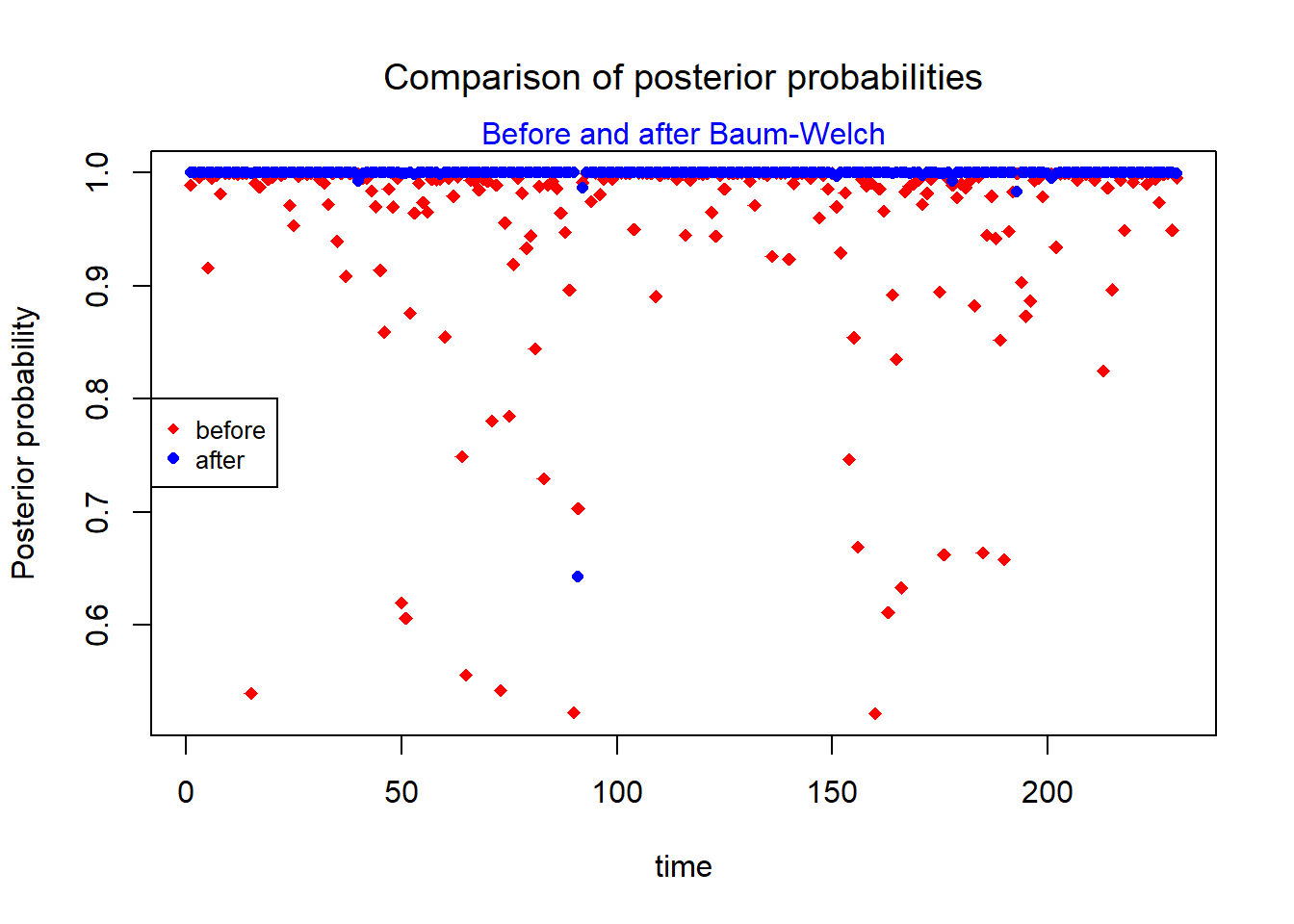Let us also compare the results of Viterbi decoding obtained before and after application of the Baum-Welch algorithm. In order to do that, we first have to carry out a Viterbi prediction as above, but considering the new parameters estimated by Baum-Welch. Therefore, we - declare the list with the new emission parameters, - create the “dthmm” model object, and - run the Viterbi: new_eparams= list(mean = bwout$pm$mean, sd = bwout$pm$sd) new_eparams ##$mean
##  2.027118 2.962835 4.044831
##
## $sd ##  0.2075000 0.3032232 0.2754277 new_model = dthmm(x = observation, Pi = bwout$Pi, delta = bwout$delta, distn = "norm", pm = new_eparams) class(new_model)  ##  "dthmm" str(new_model) ## List of 8 ##$ x       : num [1:230] 2.1 1.92 2.1 1.92 2.33 ...
##  $Pi : num [1:3, 1:3] 9.85e-01 1.01e-02 1.81e-24 1.48e-02 9.80e-01 ... ##$ delta   : num [1:3] 1.00 6.26e-22 2.51e-86
##  $distn : chr "norm" ##$ pm      :List of 2
##   ..$mean: num [1:3] 2.03 2.96 4.04 ## ..$ sd  : num [1:3] 0.207 0.303 0.275
##  $pn : NULL ##$ discrete: logi FALSE
##  $nonstat : logi TRUE ## - attr(*, "class")= chr "dthmm" new_states_predicted <- Viterbi(new_model) new_states_predicted ##  1 1 1 1 1 1 1 1 1 1 1 1 1 1 1 1 1 1 1 1 1 1 1 1 1 1 1 1 1 1 1 1 1 1 1 1 1 ##  1 1 1 2 2 2 2 2 2 2 2 2 2 2 2 2 2 2 2 2 2 2 2 2 2 2 2 2 2 2 2 2 2 2 2 2 2 ##  2 2 2 2 2 2 2 2 2 2 2 2 2 2 2 2 2 3 3 3 3 3 3 3 3 3 3 3 3 3 3 3 3 3 3 3 3 ##  3 3 3 3 3 3 3 3 3 3 3 3 3 3 3 3 3 3 3 3 3 3 3 3 3 3 3 3 3 3 3 3 3 3 3 3 3 ##  3 3 2 2 2 2 2 2 2 2 2 2 2 2 2 2 2 2 2 2 2 2 2 2 2 2 2 2 2 2 2 2 2 2 2 2 2 ##  2 2 2 2 2 2 2 2 2 2 2 2 2 2 2 1 1 1 1 1 1 1 1 1 1 1 1 1 1 1 1 1 1 1 1 1 1 ##  1 1 1 1 1 1 1 1 We still have the true state path in memory (“true_state_path”), so that we can compare the old and new predictions with the correct results. Before involving Baum-Welch, we had 14 wrong predictions: sum(states_predicted != true_state_path)  ##  14 But there is only one false prediction now: sum(new_states_predicted != true_state_path)  ##  1 which(new_states_predicted != true_state_path) ##  91 new_states_predicted ##  2 true_state_path ##  3 The only wrong prediction is at time point 91. The Viterbi predicts state 2 but the proper result would be 3. Let’s have a look at the data around this position: colvec = c(rep("blue",6), rep("darkgreen",6)) colvec = "red" plot(85:96, observation[85:96], col = colvec, type = "p", xlab = "time", ylab = "signal", pch = 16, cex = 0.85) title("Emitted signal over time (zoomed)", font.main = 1) mtext("Normally distributed signal", side = 3, col = "blue")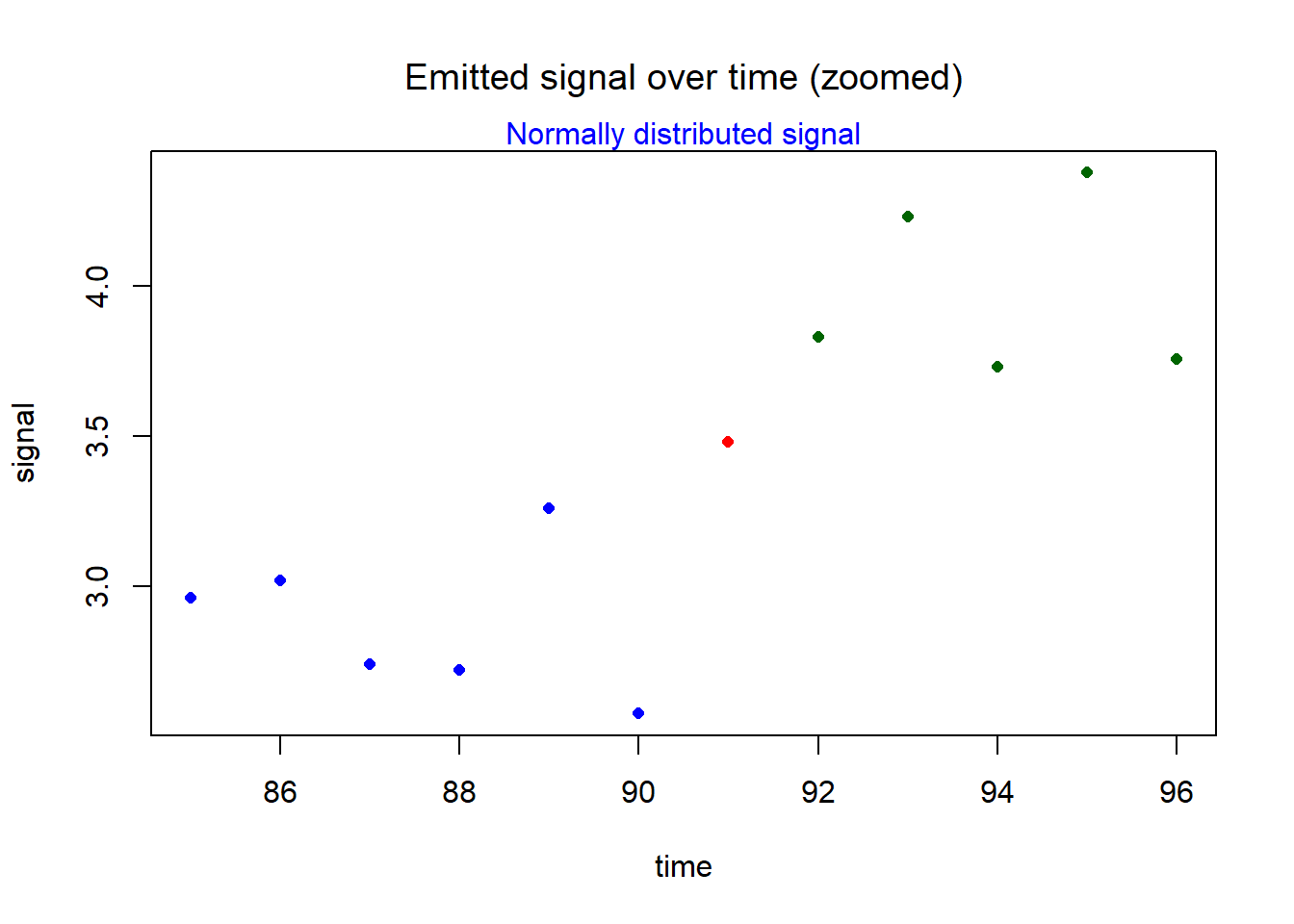The time point 91 is in red color. We see that the signal strength is relatively low considering that the true state is “EmitterC”: observation ##  3.478679 The value is closer to 3 than to 4 which is the mean for Emitter C. Moreover, the transition probability from B to C is only 1 percent: bwout$Pi[2,3]  # B-C transition probability (after BW)
##  0.01005611

As a consequence, the algorithm “thinks” that time point 91 still belongs to emitter B.

Finally, we can have a look at the likelihood (before and after Baum-Welch):

exp(Estep_out$LL)  ##  3.246657e-135 exp(bwout$LL)  
##  2.845517e-18

The likelihood has grown considerably which means that the adaption of model and data is better.
This is because the model is just better now!

The